# Create calculation puzzle

The easiest way to set up arithmetic puzzles via a hidden solution with all sorts of difficulty tweaks. Make calculation exercises even more fun!## The calculation puzzle maker

Creating puzzles with numbers is normally not as straightforward as with text, but now it is! Setting up puzzles around mathematical operations is super easy with the calculation puzzle maker, and even better, it has a hidden solution as the basis!

Creating and solving the numbers puzzle is now fun, user-friendly and educational. It will generate one exercise per character in your hidden solution, which the player then needs to connect to an outcome by just selecting the calculation and the outcome that belong together.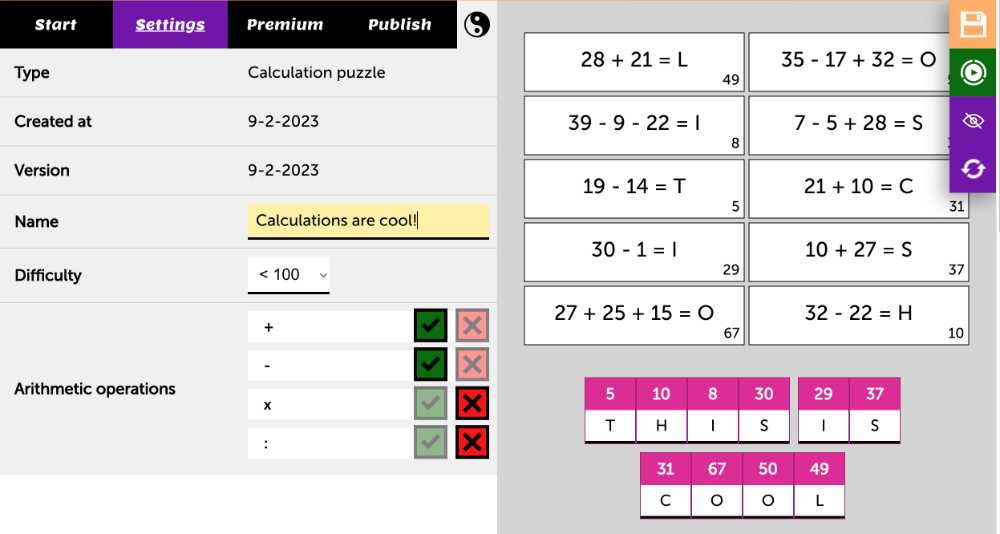To start off, just enter a sentence on the Start-tab and the calculation puzzle algorithm does the rest!

The exercises that are generated are based on several settings that you can tweak to get the perfect repertoire for your students.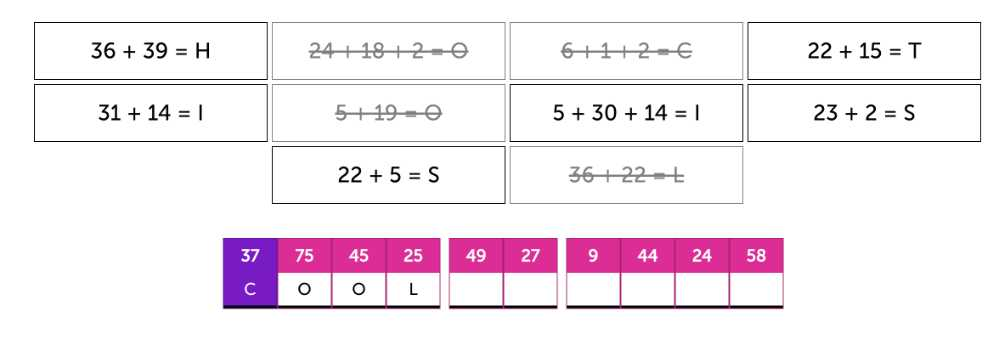## Tweaking the difficulty

Since students of all ages can use the calculation puzzle tool, having enough options to determine the difficulty is important.

The first way to account for this, is by choosing the maximum number for the outcome of a calculation. This will heavily impact the types of calculations that you will get since the lower the number gets, the less freedom the algorithm has.

Note that picking '< 20' here, limits the hidden solution to 20 characters since each outcome needs to be unique (otherwise the puzzle would not work).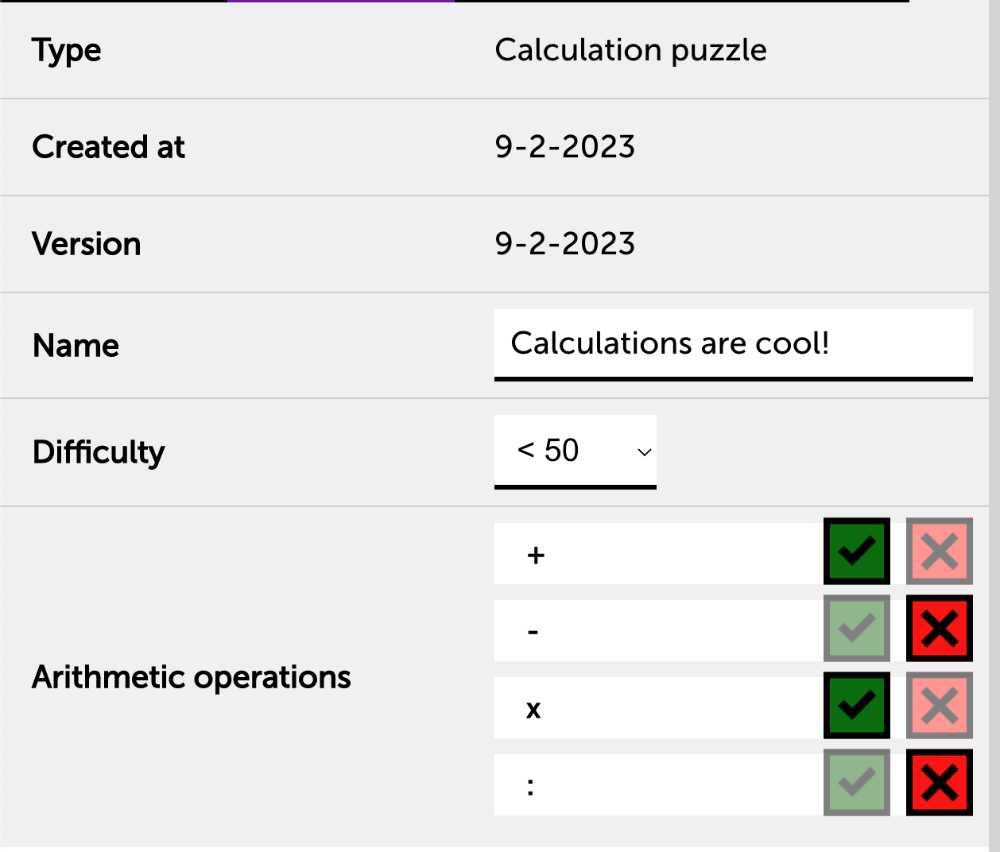## Picking the type of operations

Since the difficulty is also determined by the type of operations, you can handpick which ones you want with ease.

Dividing and multiplication is cool of course, but sometimes a good old add and subtract exercise can be just as educational and fun :).## Making the calculations more challenging

An interesting way to crank up the difficulty, is by changing the 'Maximum number of operations' setting on the Premium-tab to 2 or 3. This way, multiple numbers and operations are combined and this will challenge the player to insert more calculating skills.

Note that the algorithm keeps the precedence of the operations in mind, so multiplication and division will never come after addition and subtraction, this was done to keep it clear for the player.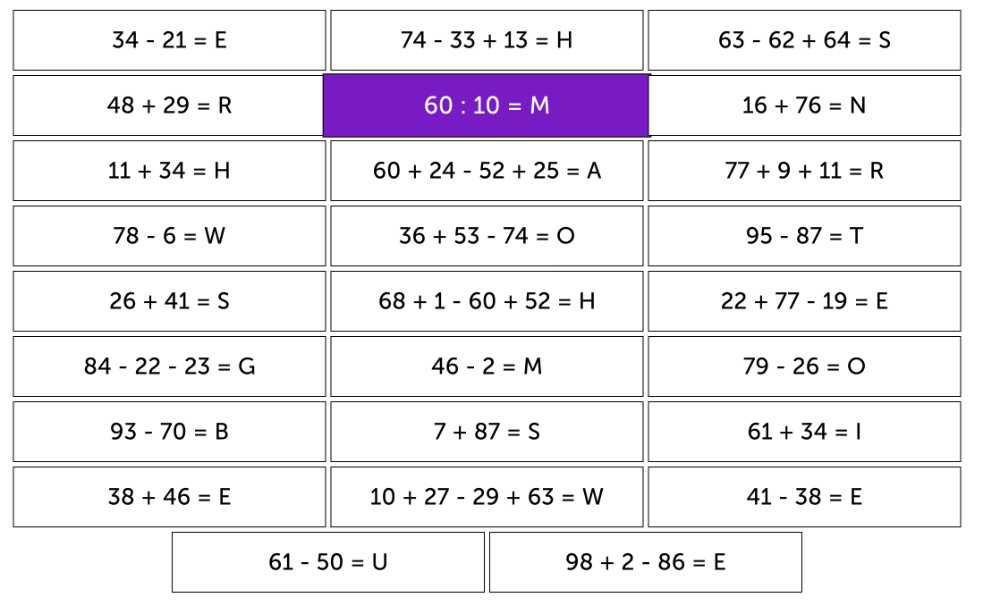## Customise the types of calculations

Since every teacher has own goals during their classes, it might come in handy to tweak the types of calculations even further.

By tweaking the 'Highest number to use' setting on the Premium-tab to '10' for example. This will create the classic multiplication table calculations for younger students.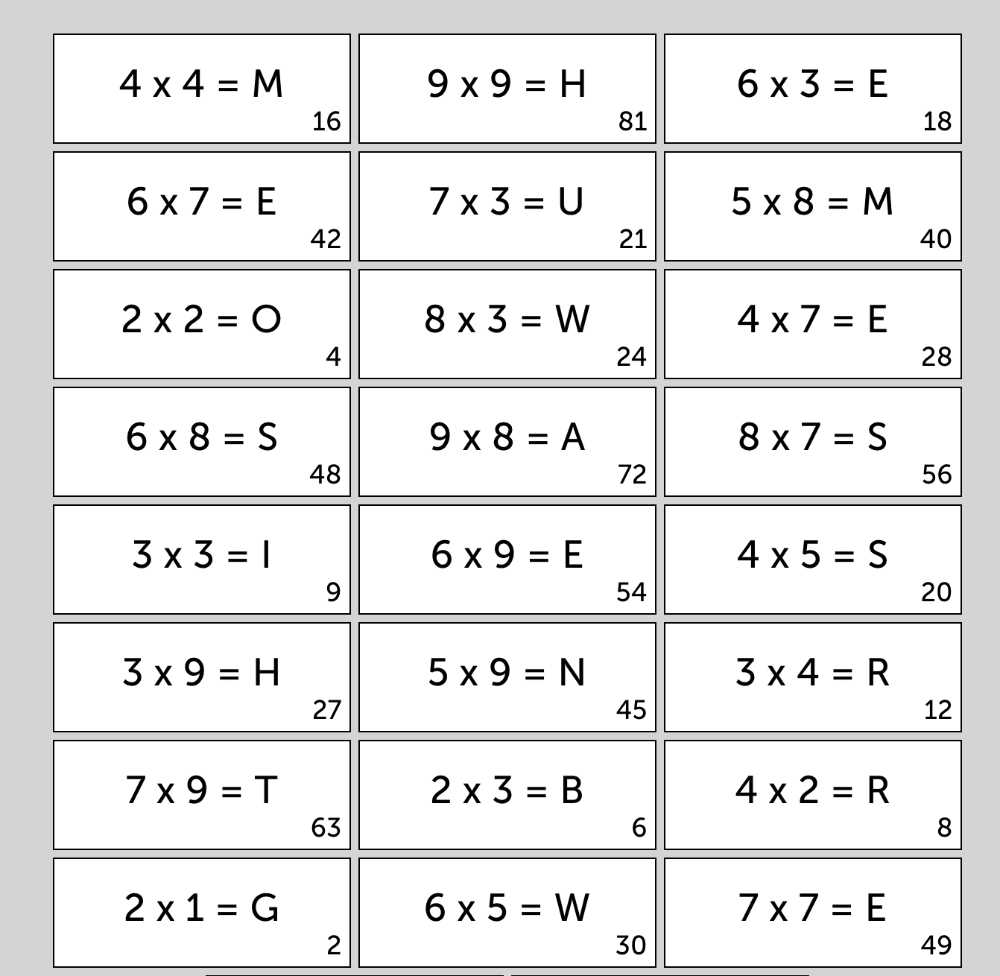## Check out the sample puzzle

Discover how this puzzle type looks in practice by viewing and playing the sample puzzle (opens in a new tab)